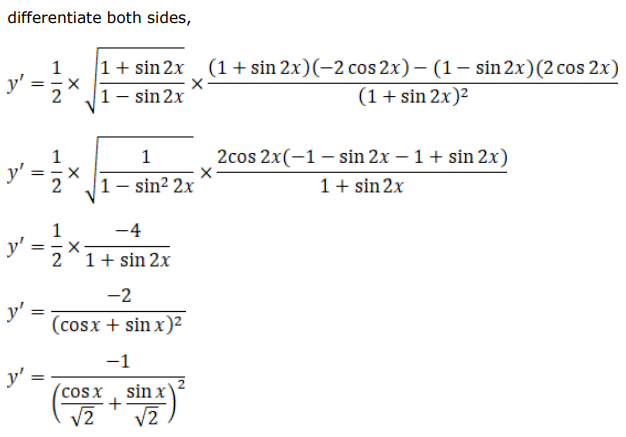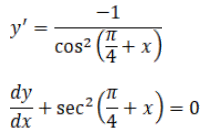# Solve this followingQuestion:

If $y=\sqrt{\frac{1-\sin 2 x}{1+\sin 2 x}}$, show that $\frac{d y}{d x}+\sec ^{2}\left(\frac{\pi}{4}-x\right)=0$

Solution: